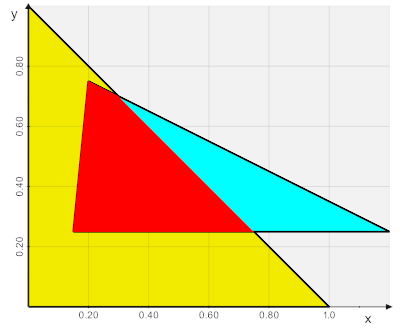# 2D Triangle intersection

I wonder if there is a way of finding the overlapping area of two arbitrary triangles in 2D.

I could only come up with the idea of extruding the 2 triangles (`vtkRotationalExtrusionFilter`) and find the intersection with `vtkBooleanOperationPolyDataFilter`
It actually works (see below using `vtkplotter`), but i guess there must be a more clever way of doing it.

``````from vtkplotter import *

verts1 = [(0,0), (1,0),(0,1)]
verts2 = [(0.15,0.25),(1.2,0.25),(0.2,0.75)]
faces  = [(2,1,0)]

# Create an extrusion surface along positive z
a1  = Actor([verts1, faces]).z(-0.02).lw(2).c('gold')
ae1 = extrude(a1, zshift=0.04)

a2  = Actor([verts2, faces]).z(-0.01).lw(2).c('aqua')
ae2 = extrude(a2, zshift=0.02)

# Intersection in 3D
a3 = booleanOperation(ae1, "intersect", ae2).c('r').flat()

# Project 3D object on a z=cost plane by setting z coords
a3.coordinates()[:,2] = 0
area = a3.area() / 2 # divide by 2 because of back face

print('Area is', area)
show(a1, a2, a3, bg='white', axes=1)
``````